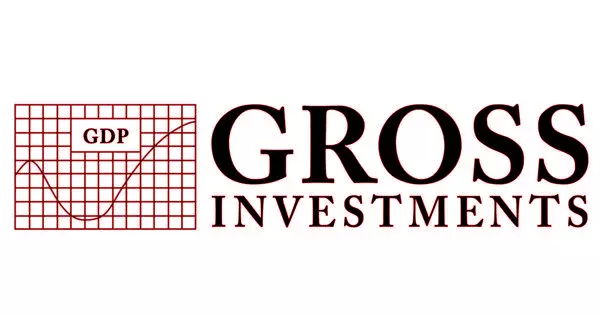Economics

# Gross Investment – in EconomicsThe total expenditure or investment made by a company to acquire capital goods is defined as gross investment. It is the total amount spent by the economy on new capital. This figure includes an estimate of the value of capital depreciation, because some investment is required each year simply to replace technologically obsolete or worn-out plant and machinery.

The gross investment is the gross value of such an expenditure that does not account for depreciation (which is wear and tear of an asset over its useful life). It consists of gross fixed investment plus net stock and work-in-progress investment. It is distinguished from net investment, which measures the change in the capital stock after allowing for capital consumption.

Net investment is calculated by subtracting the value of depreciation from the gross investment. If the value of gross investment is greater than the value of depreciation at any given time, it indicates that net investment is positive and the capital stock has increased. However, gross investment does not reflect the actual change in the economy’s stock of productive assets over a given year. Some fixed capital is consumed during the manufacturing process. Depreciation is the loss of fixed capital. Net investment is calculated by subtracting depreciation from gross investment.

Calculation

Example: A company’s gross investment is the amount it has invested in an asset or business before depreciation. When depreciation is taken into account, net investment is created. For example, suppose a company spends \$5,000 on a car that depreciates by \$3,000 after three years. The gross investment in year three is \$5,000, and the net investment is \$2,000. This is important for tracking how much money was actually spent on the investment. This calculation is also used in business formulas such as cash return on gross investment.

• Step 1 – Find the asset on the company’s balance sheet. For example, the company has property valued at \$500,000 on the balance sheet.
• Step 2 – Find the accumulated depreciation on the company’s balance sheet. In the example, the property has \$200,000 of accumulated depreciation.
• Step 3 –Add the accumulated depreciation to the company’s book value of the asset to find the gross investment in the asset. In the example, \$500,000 plus \$200,000 equals a gross investment of \$700,000.

Similarly, if the gross investment value is less than the depreciation, the net investment becomes negative, resulting in a decrease in capital stock. It is based on observable market transactions; capital consumption, on the other hand, is based on calculations about the rate at which capital goods wear out or become obsolete.